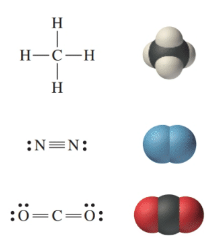# The Lewis structures for CH 4 , N 2 , and CO 2 , along with the corresponding space-filling molecular images, are shown here: Use Lewis theory and VSEPR theory to draw similar space-filling molecular images of a . CO b . NH 3 c . H 2 S d . SiH 4### Chemistry In Focus

7th Edition
Tro + 1 other
Publisher: Cengage Learning,
ISBN: 9781337399692

#### Solutions

Chapter
Section### Chemistry In Focus

7th Edition
Tro + 1 other
Publisher: Cengage Learning,
ISBN: 9781337399692
Chapter 5, Problem 45E
Textbook Problem
212 views

## The Lewis structures for CH 4 , N 2 , and CO 2 , along with the corresponding space-filling molecular images, are shown here:Use Lewis theory and VSEPR theory to draw similar space-filling molecular images of a . CO b . NH 3 c . H 2 S d . SiH 4

Interpretation Introduction

Interpretation:

The space-filling molecular images using the Lewis and VSEPR theories are to be drawn.

Concept Introduction:

 The Lewis dot symbols contain dots, which give information about valence electrons.

 The Lewis dot symbols show both bonds and lone pairs as dots.

 The Lewis structures show bonds as lines and lone pairs as dots.

### Explanation of Solution

a) CO

The number of valence electrons in CO is calculated as follows:

=(C atoms)+(O atom)=4+6=10

Now, only six electrons are used in bond formation to complete the octet of carbon and oxygen. So, the number of remaining electrons is four and there is one lone pair present on carbon and one on oxygen.

Therefore, the Lewis structure of CO is:

There are three bond pairs and two lone pairs. So, by VSEPR theory, the shape is linear because only two atoms are involved. So, the space-filling molecular image can be formed as follows:

b) NH3

The number of valence electrons in NH3 is as follows:

=(N atoms)+3(H atom)=5+3=8

Only six electrons are used in bond formation to complete the octet of nitrogen and hydrogen. Therefore, the number of remaining electrons is two and there is one lone pair present on nitrogen.

Hence, the Lewis structure of NH3 is:

There are three bond pairs and one lone pair. So, by VSEPR theory, the shape is tetrahedral because only four atoms are involved. So, the space-filling molecular image is as follows:

### Still sussing out bartleby?

Check out a sample textbook solution.

See a sample solution

#### The Solution to Your Study Problems

Bartleby provides explanations to thousands of textbook problems written by our experts, many with advanced degrees!

Get Started

Find more solutions based on key concepts
Why do we use different map projections?

Fundamentals of Physical Geography

Energy-yielding nutrients include all of the following except. a. vitamins b. carbohydrates c. fat d. protein

Nutrition: Concepts and Controversies - Standalone book (MindTap Course List)

Describe what happens to the level of blood lipids after a meal rich in fat.

Chemistry for Today: General, Organic, and Biochemistry

Why does helium fusion require a higher temperature than hydrogen fusion?

Horizons: Exploring the Universe (MindTap Course List)

A sample of lead has a mass of 20.0 kg and a density of 11.3 X 103 kg/m3 at 0C. (a) What is the density of lead...

Physics for Scientists and Engineers, Technology Update (No access codes included)

What is a fully developed sea?

Oceanography: An Invitation To Marine Science, Loose-leaf Versin